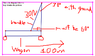# Force Problem, Constant F=30N , 30*angle with ground, d=100m

• Steb
In summary, the child pulls a wagon with a constant force of 30 N at a distance of 100 m. The work done in moving the wagon is 1500 J.f

#### Steb

1.Homework Statement
A child pulls a wagon with a constant force of 30 N. How much work is done in moving the wagon 100 m if the handle makes an angle of 30* with the ground?

## Homework Equations

Brown = Vector
W=fd

a2+b2=c2

sinθ= Opposite/Hypotenuse

## The Attempt at a Solution

I calculated the force on the x-axis of the triangle since the 100m distance also lies on the x axis.

cos60*=x/30
x=15

I got an answer of 15N for the magnitude of the force and sub this into the work formula along with the 100m distance.

W=fd
W= (15)(100)
W= 1500J

Geometry and Discrete Mathematics 12 states the answer to be approximately 2600J. The question is #21 on page 58 of the book. What is the correct approach to solve this problem?

#### Attachments

•Capture.PNG
11.8 KB · Views: 369
Hello Steb,

Welcome to Physics Forums!

Your approach is correct, but not your interpretation of the problem. The crux of the "mistake" is the interpretation of
...the handle makes an angle of 30* with the ground...
That angle isn't supposed to be relative to a line perpendicular to the ground, but rather a line parallel to the surface of the ground.

In other words, in your block diagram, change the place where you had 30o to 60o, and the place where you had 60o to 30o.[Edit: an easy way to remember this for future, similar problems, is that the force in the direction of the handle is the hypotenuse, and it is 30o with respect to the ground. That 30o angle is where the handle and ground meet. Knowing that, the vector parallel to the ground is the adjacent side of the triangle (with respect to the 30o angle).]

[Another edit: When talking about a "Normal" force, the vector in question is typically perpendicular to the surface. So in those cases, yes, the vector is perpendicular to the surface. However, this problem doesn't involve the normal force, and the way the problem statement was phrased, it did not imply an angle of 30o with respect to the normal; rather it implied 30o with respect to the surface (parallel).]

Last edited:
Hello Steb,

Welcome to Physics Forums!

Your approach is correct, but not your interpretation of the problem. The crux of the "mistake" is the interpretation of

That angle isn't supposed to be relative to a line perpendicular to the ground, but rather a line parallel to the surface of the ground.

In other words, in your block diagram, change the place where you had 30o to 60o, and the place where you had 60o to 30o.[Edit: an easy way to remember this for future, similar problems, is that the force in the direction of the handle is the hypotenuse, and it is 30o with respect to the ground. That 30o angle is where the handle and ground meet. Knowing that, the vector parallel to the ground is the adjacent side of the triangle (with respect to the 30o angle).]

[Another edit: When talking about a "Normal" force, the vector in question is typically perpendicular to the surface. So in those cases, yes, the vector is perpendicular to the surface. However, this problem doesn't involve the normal force, and the way the problem statement was phrased, it did not imply an angle of 30o with respect to the normal; rather it implied 30o with respect to the surface (parallel).]
Thanks